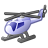# How to determine the coordinates of an object on the road, if it is known that it is located at a certain distance from the beginning of the road?

3811
4
02-02-2015 05:38 AMOccasional Contributor III

Dear Gurus!

How to determine the coordinates of point on the road (on the client side), whose distance from the beginning of the road (the distance is measured along the road, not the shortest distance from point to point)?

P.S. Should I write a service that performs this task, or are there other options?

1 Solution

Accepted SolutionsbyEsri Frequent Contributor
4 Repliesby
Regular Contributor

HI Anatollii,

You have to options:

1) Use Roads&Highways extension to ArcGIS For Server. Use the sample below

2) Do some azimuth and length calculations.

.

1 case) The route is straight (line):

Calculate route length (Dpk) using coordinates of starting (P) and ending (K) point of the route. Calculate         deltaXpk = Xk – Xp and delta Ypk = Yk – Yp. Get your known length Dpn

Coordinates of the point are: Xn = Xp + (Dpn x deltaXpk) / Dpk and  Yn = Yp +(Dpn x deltaYpk)) / Dpk

2 case) The route is not straight (polyline):

If  Dpn > Dpk, change the points. Point P = Point K. Point K equals next vertex on the polyline. Check the distance if Dpn > Dpk + Dpk(of previous sections). If true, go to next section. If false, calculate coordinates.

Regards,byEsri Frequent ContributorOccasional Contributor III

Excellent!New Contributor III

For me I would:

Use Latitude and departures as long as you are using something like state plane coordinates.

1) get coordinate of start of road.

2) determine the azimuth of the road 0 - 359.9999 degrees

3) Cos of the azimuth * the distance from beginning of road is what is called the Latitude (algebraic difference in Y).

4) Sin of the Azimuth * the distance from the beginning of the road is what is called the departure (algebraic difference in X)

5 ) Add the latitude (keeping the sign in tact) to original Y coordinate from step 1

Note Latitude mentioned above is not a geographic Latitude.

In example above calculator set to Degrees mode and not Radians.

or

Create a line feature class.

Go into edit mode and add a line with the first vertex of line being the beginning of the road.

Then moving mouse to a point on centerline of the road somewhere beyond where you think the point will be and then right click and pick direction and just accept the bearing that it shows.  Then right lick and pick distance and enter the distance.  If you id the end of the line you just drew you will have your coordinate.